# Funky 8 Carpet Fractal

For my fractal, I removed the coordinates [0,0], [2,1], [1,2], and [3,3].  This created a shape that is symmetrical and that is why I like it.  If you turn it a little, it looks like the number ‘8’ but with straight lines instead of curved ones.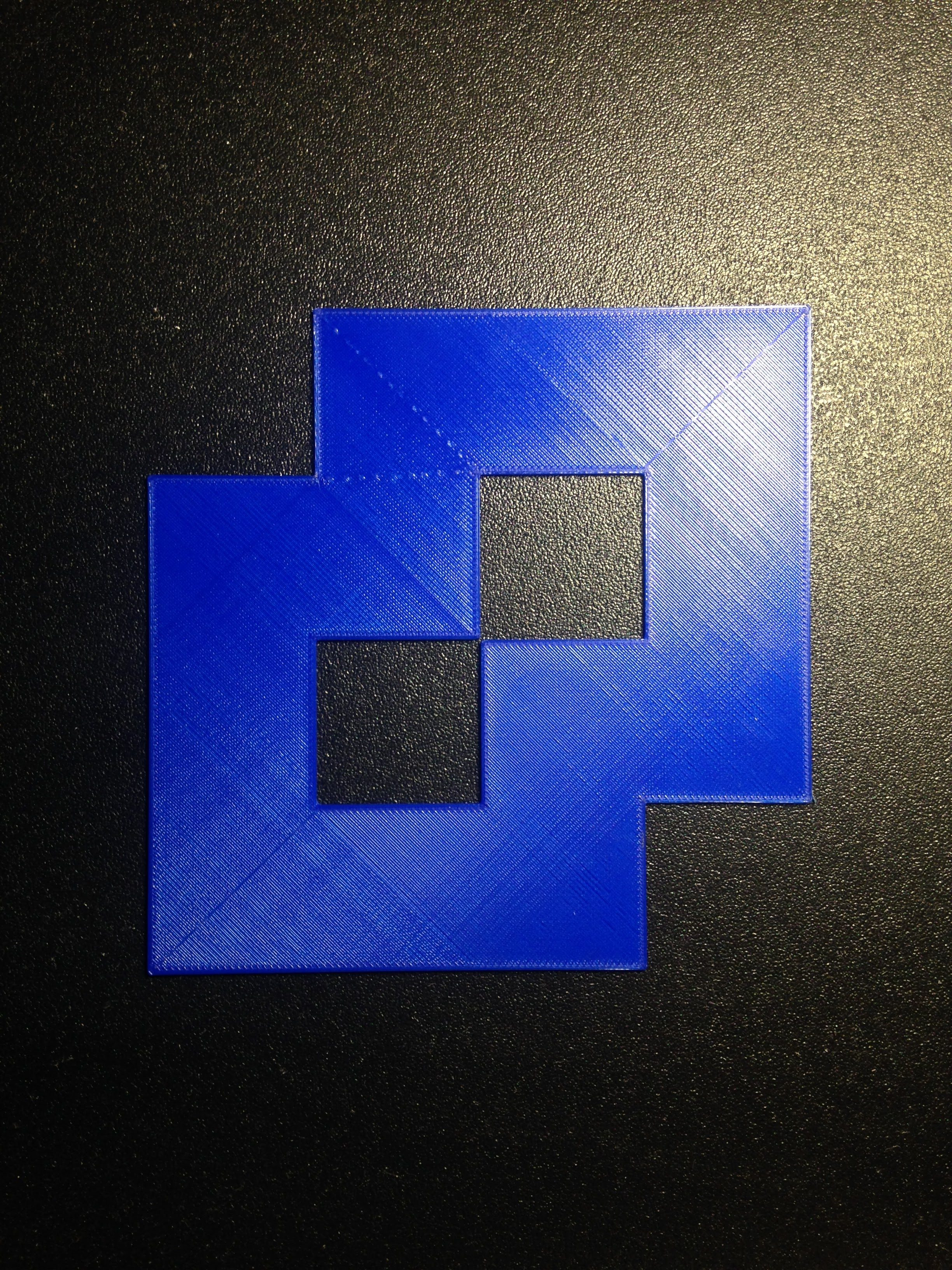### Area Calculations

Level 1:
For this fractal, we assumed the area 16 units.  Each large square we divided it into has the area of 1 unit.  I removed 4 squares so the area for my level 1 fractal is

(area of the original carpet fractal) – [(area of each large square removed) x (number of large squares removed)]
or
16 – (1×4) = 12.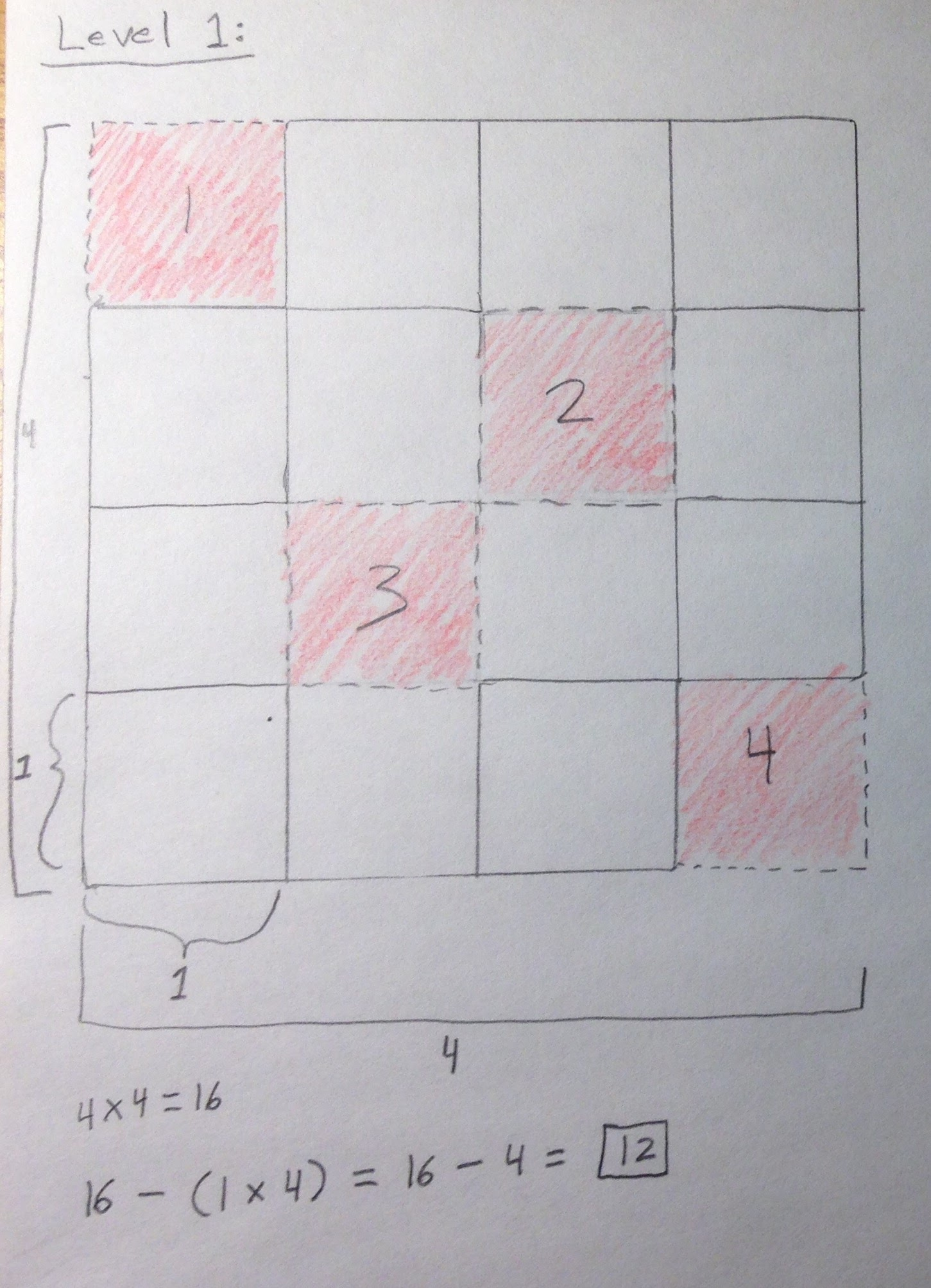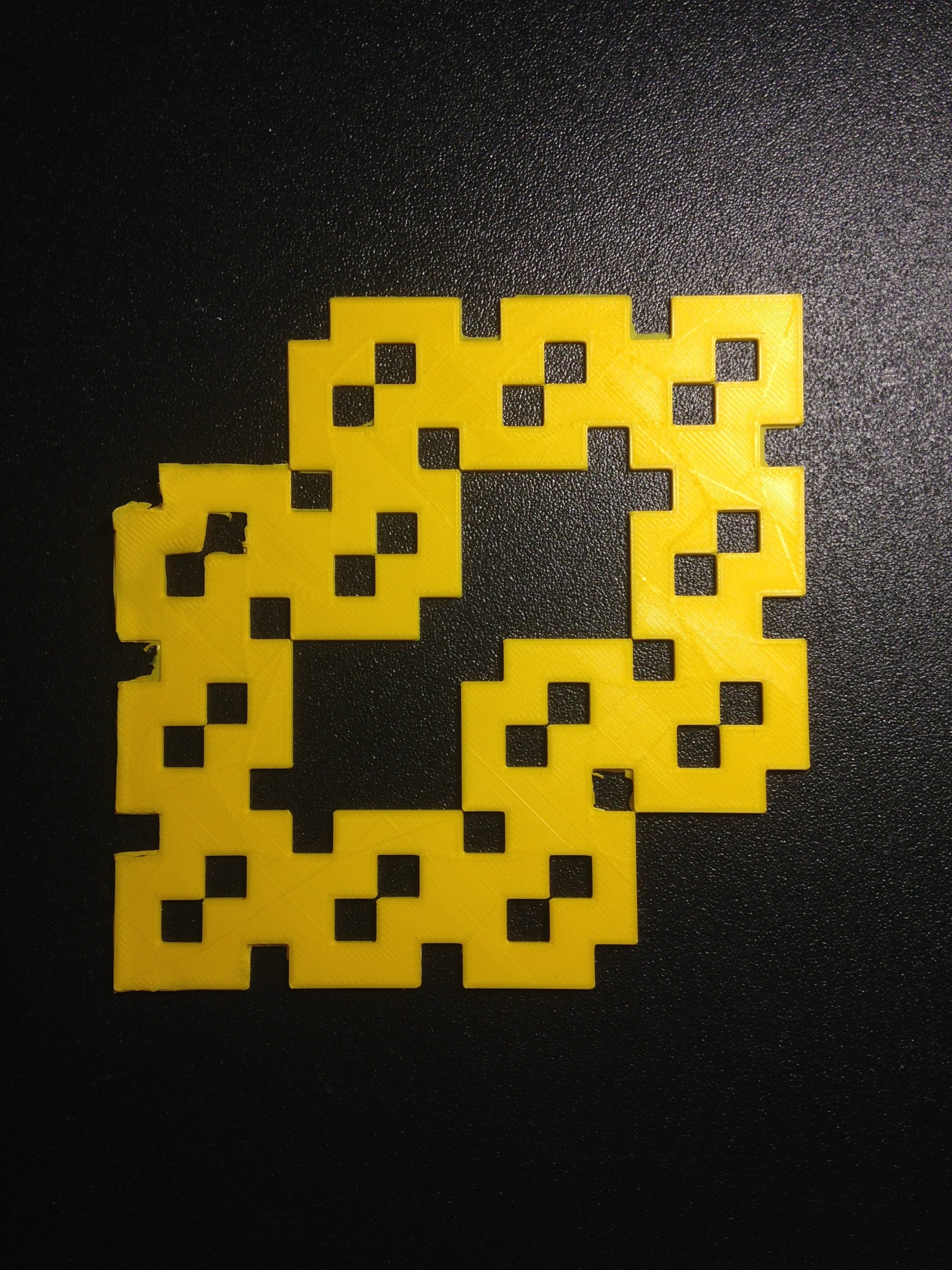Level 2:
For the area of level 2, I took the area of level 1 ([16 – (1 x 4)] or 12) and subtracted the area of a medium sized square (1/16) times the number of medium sized squares removed (48).

(area of the level 1) – [(area of each medium square removed) x (number of medium squares removed)]
or
12 – [(1/16) x 48] = 9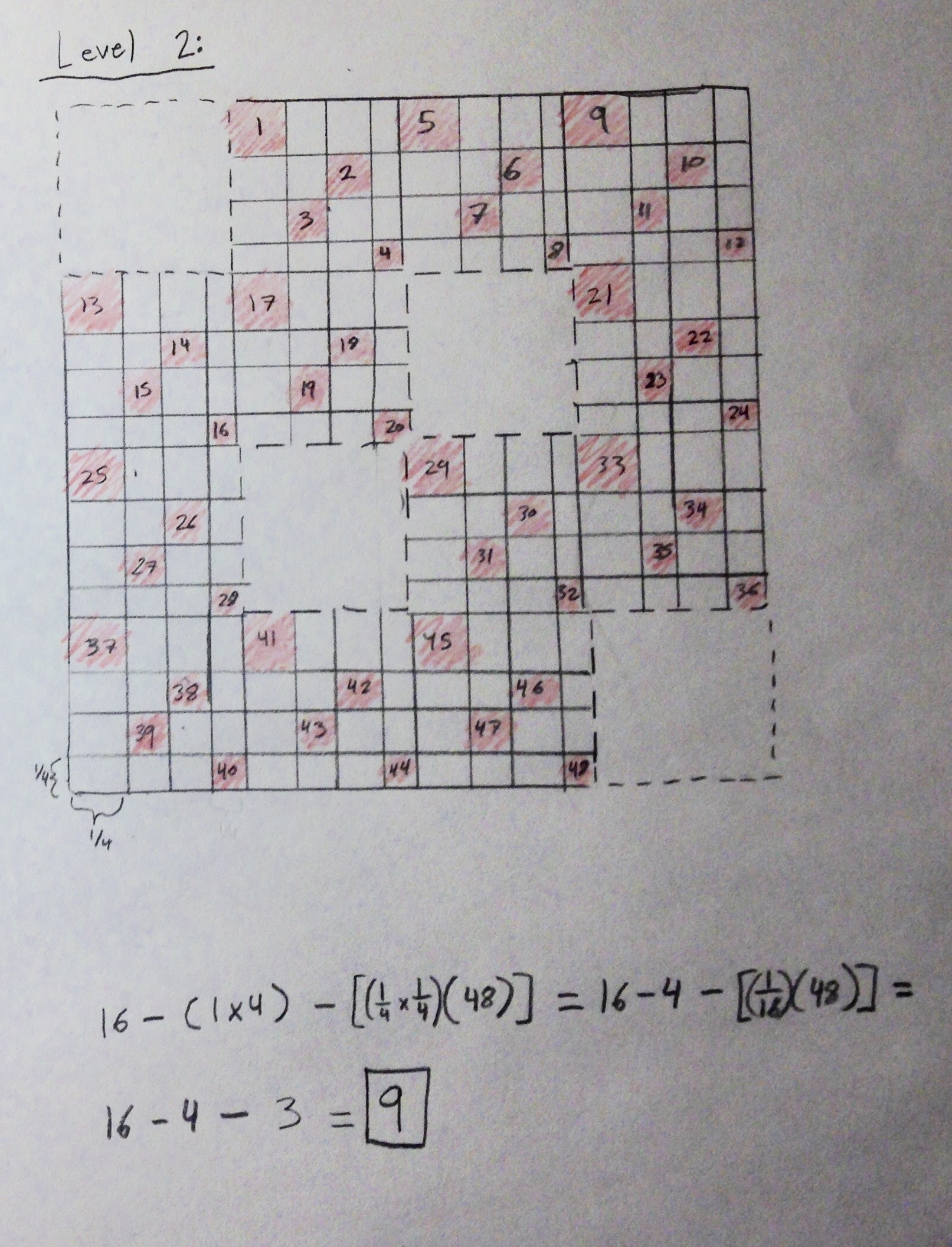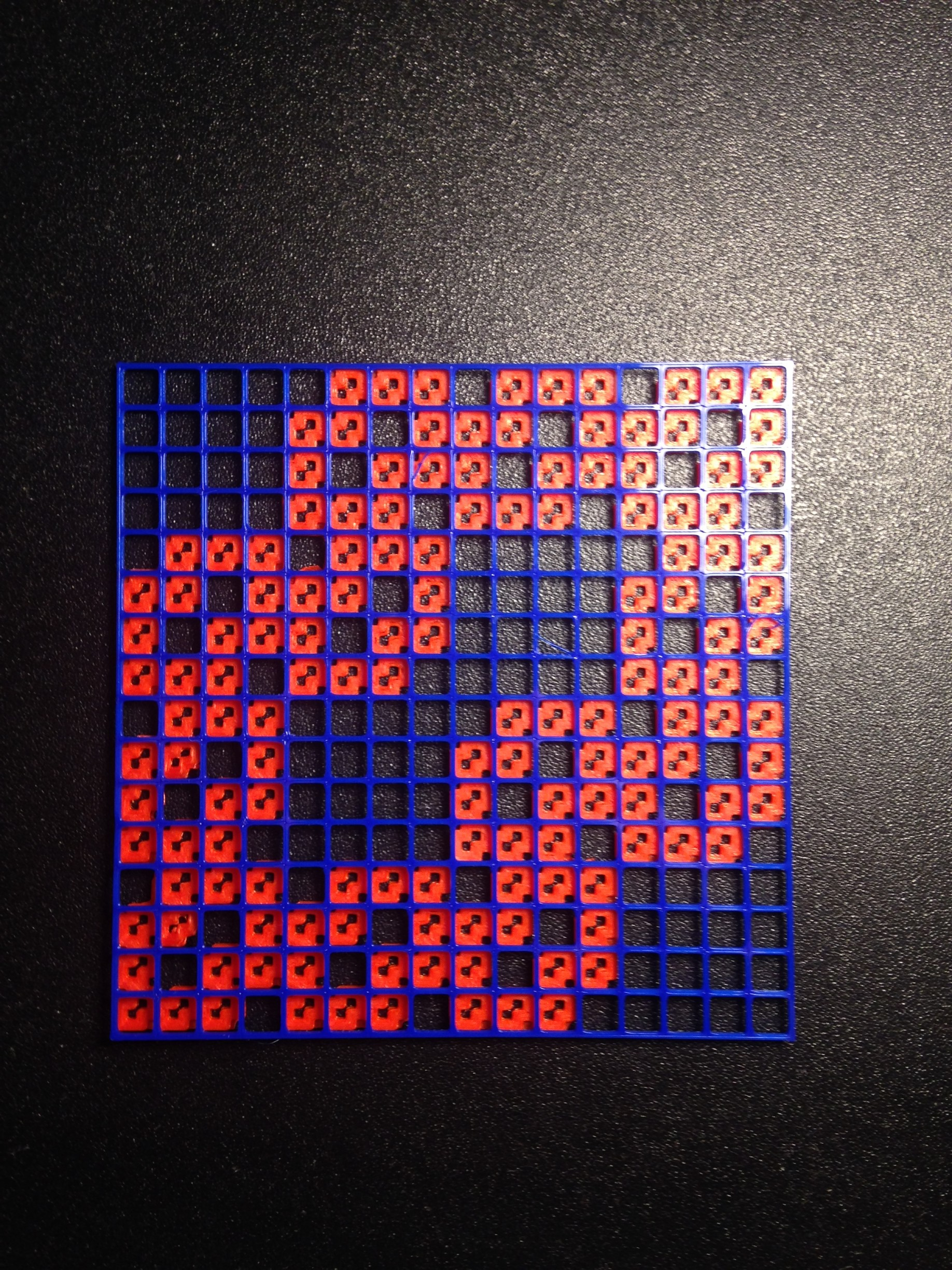Level 3:
For the area of my level 3 fractal, I took the area of level 2 (12 – (1/16 x 48) or 9) and subtracted the area of a small sized square (1/256) times the number of small sized squares removed (576).

(area of level 2) – [(area of each small square removed) x (number of small squares removed)]
or
9 – [(1/256) x 576] = 6.75

### Infinite Geometric Series Calculations

To calculate the geometric series, we use this formula: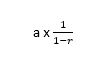To use it, I need to know what ‘a’ and ‘r’ are.
First, I will use this to help understand where ‘a’ and ‘r’ are coming from: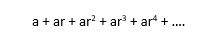Now I will write out the area calculations all together like this: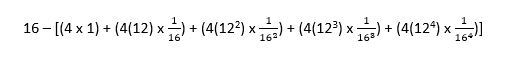Next, I re-wrote it so that all the terms with a power were together like this: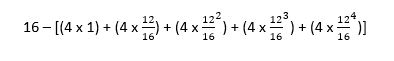Now we can see which number is repeated and which number has the power like this one:We can see that 4 is the number that is repeated and 12/16 is the number to the power so:
a = 4
r = 12/16

Now I can use the formulaand plug in 4 for a and 12/16 for r to get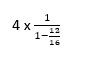which can me simplified to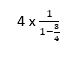We solve and end up with 4 x 4 which is 16 which is surprising because that is the area of our original carpet fractal.

### Dimension Calculations

To find the dimensions, we need to look at the linear scaling factor and how many of the scaled down versions of the fractal make up the whole fractal.  The linear scaling factor is how many pieces the bottom line is divided into.  In this case, the linear scaling factor is ¼.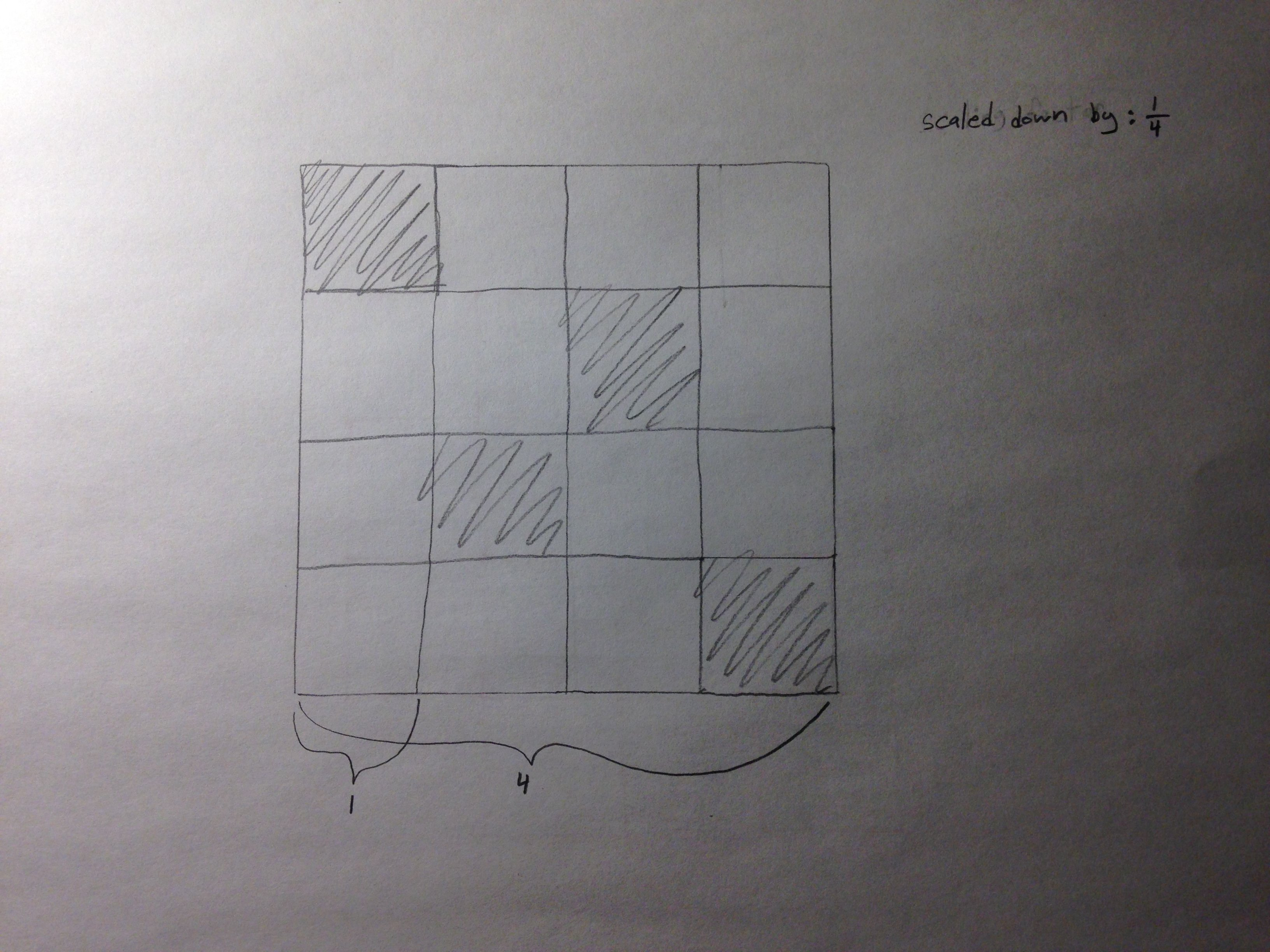The other number we need is how many smaller copies make up the larger whole fractal.  In this case that number is 12.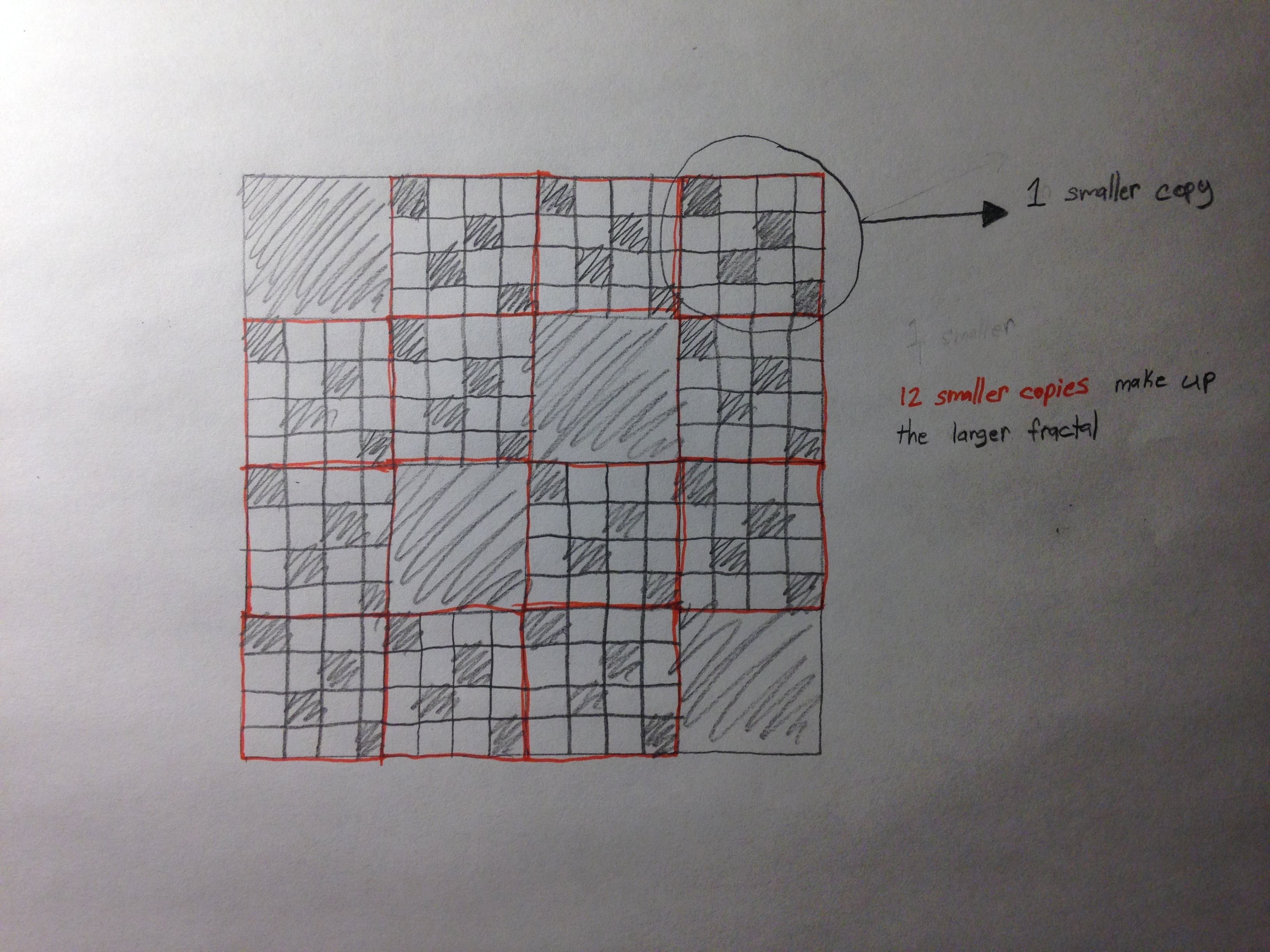Then, I take the formula for finding dimension: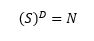S is the scale down factor, D is dimension, and N is the number of smaller copies that make up the larger fractal.  I plug in the numbers and get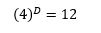Then, I have to solve for dimension (D).  For this I use Log and get: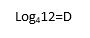I plugged that into my calculator and got

D = 1.79

so the dimension of my fractal is 1.79, neither 1 nor 2 dimensions.

Sharing:
Here is a link to my fractal on Thingiverse.